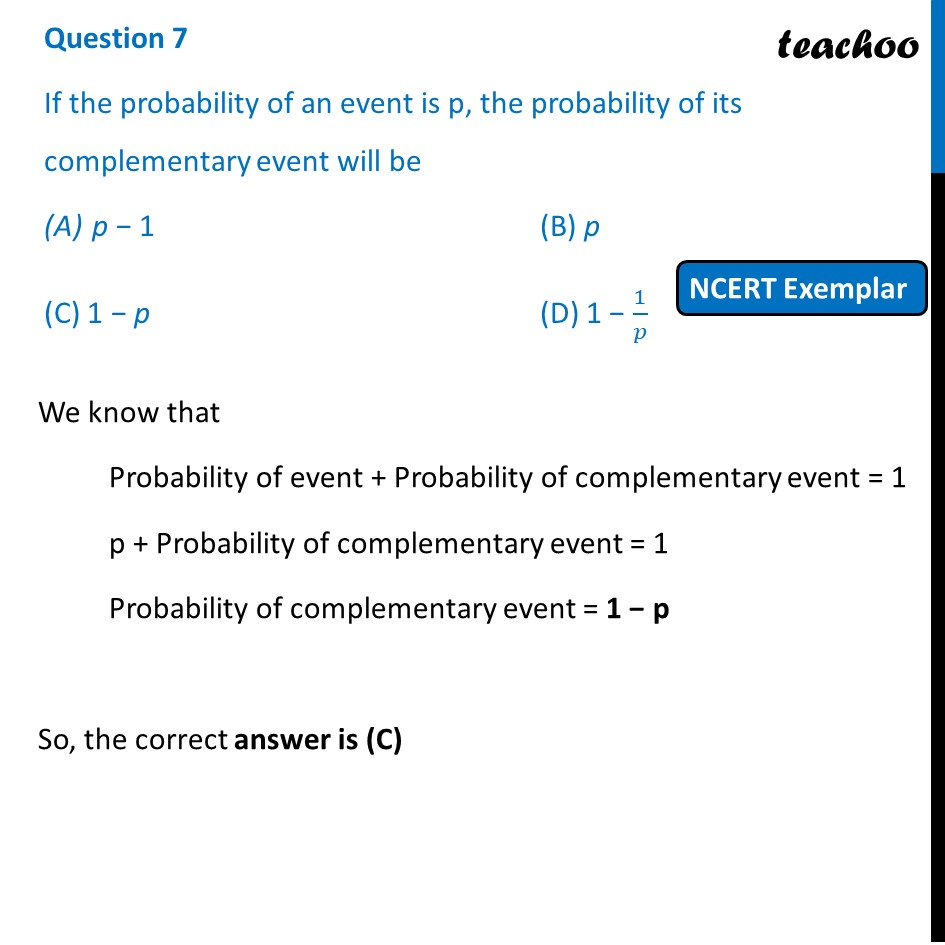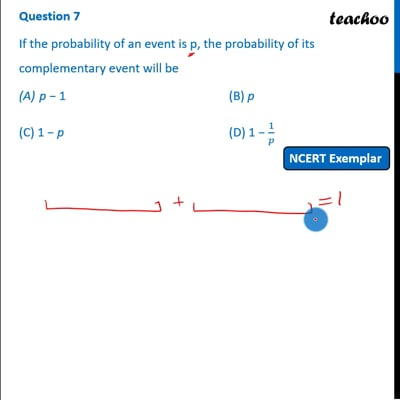NCERT Exemplar - MCQ

Chapter 15 Class 10 Probability
Serial order wise

## (D) 1 − 1/pThis video is only available for Teachoo black users

Introducing your new favourite teacher - Teachoo Black, at only ₹83 per month

### Transcript

Question 7 If the probability of an event is p, the probability of its complementary event will be p − 1 (B) p (C) 1 − p (D) 1 − 1/𝑝 We know that Probability of event + Probability of complementary event = 1 p + Probability of complementary event = 1 Probability of complementary event = 1 − p So, the correct answer is (C)